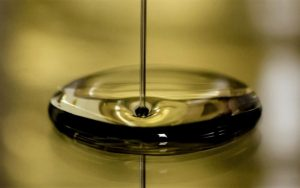Themes

# What is the Newtonian fluid?

## Newtonian fluid

A fluid is said to be Newtonian if its viscosity , which is the measure or ability of a fluid to resist flow , only varies as a response to changes in temperature or pressure . A Newtonian fluid will take the shape of its container. Under conditions of constant temperature and pressure, the viscosity of a Newtonian fluid is the constant of proportionality , or the relationship between the shear stress that is formed in the fluid to resist flow and the shear rate applied to the fluid to induce it; the viscosity is the same for all speedsshear applied to the fluid. Water, sugar solutions, glycerin, silicone oils, light hydrocarbons, air, and other gases are all examples of Newtonian fluids. Most drilling fluids are non-Newtonian fluids.## What is the Newtonian fluid?

The Newtonian fluid is a fluid that has a viscosity that can be considered constant over time with a curve that shows the relationship between the stress versus the strain rate is linear .

• Newtonian fluid characteristics
• Classification of Newtonian fluids
• Newtonian fluid viscosity
• Temperature
• Newtonian Fluid Examples

## Newtonian fluid characteristics

The main characteristics of the Newtonian fluid are the following:

Related Articles
• Newtonian fluids do not have any elastic properties .
• They are incomprehensible , they are isotropic and unreal .
• Viscosity is temperature dependent .
• The viscosity also depends on the different pressures in which it is.
• When they are at a fixed temperature , their viscosity does not change and remains constant.
• These fluids are said to have a normal behavior , in which there is very little viscosity and it does not vary with forces that are applied on it.
• As the temperature increases in a fluid, so does its viscosity .
• The viscosity of the liquid is inversely proportional to the increase in its temperature.
• The Newtonian fluid was named by Isaac Newton , who described it as a viscous flow.
• They comply with Newton’s law of viscosity.

## Classification of Newtonian fluids

Newtonian fluids can be classified depending on the relationship between the stress shear applied to the flow and speed of deformation resulting from this effort. The fluids in which the stress is shear are directly proportional to the strain rate, and are called Newtonian fluids.

## Newtonian fluid viscosity

The study carried out with respect to the different types of viscosity of liquids is based on studying the different velocity profiles that are generated under certain types of conditions, always considering first the Newtonian idea, in which viscosity is a constant that it will depend mainly on the temperature and to a lesser extent it will depend on the pressure .

## Temperature

In a fluid that has already been considered a Newtonian fluid, the viscosity will depend solely on the temperature and the differences that are present. As the temperature increases , thus the viscosity of the fluid will decrease . By this we mean that the viscosity of fluids is inversely proportional to the increase in temperature.

## Newtonian Fluid Examples

There are a large number of fluids that have the necessary characteristics to be considered a Newtonian fluid , in addition to behaving like a Newtonian fluid under normal conditions of pressure and temperature . Among them we can mention the following:

SAE 30 oil, which is an engine oil and transition is used at usually in cars.

All those foods that show ideal flow characteristics , some of them of great physiological importance, such is the case of water , milk of any type of animal that can produce it, and drinks that are taken as refreshing .

All those solutions that are made with sugars , therefore, all types of drink carbonated drinks, alcoholic not containing long chain molecules, the syrups of corn and some honeys fall into this classification.

• Hydrogen.
• The gasoline .
• The air.
• The different types of oils that exist.
• Ethyl alcohol.
• The mercury .
• Glycerin.
• Benzene.
• All those compound liquids based on oils.